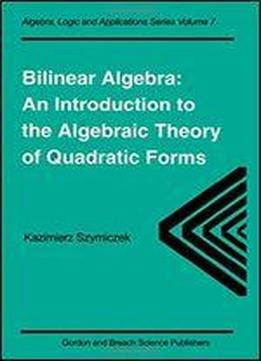# Bilinear Algebra: An Introduction To The Algebraic Theory Of Quadratic Forms by Kazimierz Szymiczek / 1997 / English / PDF

Giving an easily accessible elementary introduction to the algebraic theory of quadratic forms, this book covers both Witt's theory and Pfister's theory of quadratic forms. Leading topics include the geometry of bilinear spaces, classification of bilinear spaces up to isometry depending on the ground field, formally real fields, Pfister forms, the Witt ring of an arbitrary field (characteristic two included), prime ideals of the Witt ring, Brauer group of a field, Hasse and Witt invariants of quadratic forms, and equivalence of fields with respect to quadratic forms. Problem sections are included at the end of each chapter. There are two appendices: the first gives a treatment of Hasse and Witt invariants in the language of Steinberg symbols, and the second contains some more advanced problems in 10 groups, including the u-invariant, reduced and stable Witt rings, and Witt equivalence of fields.

views: 375